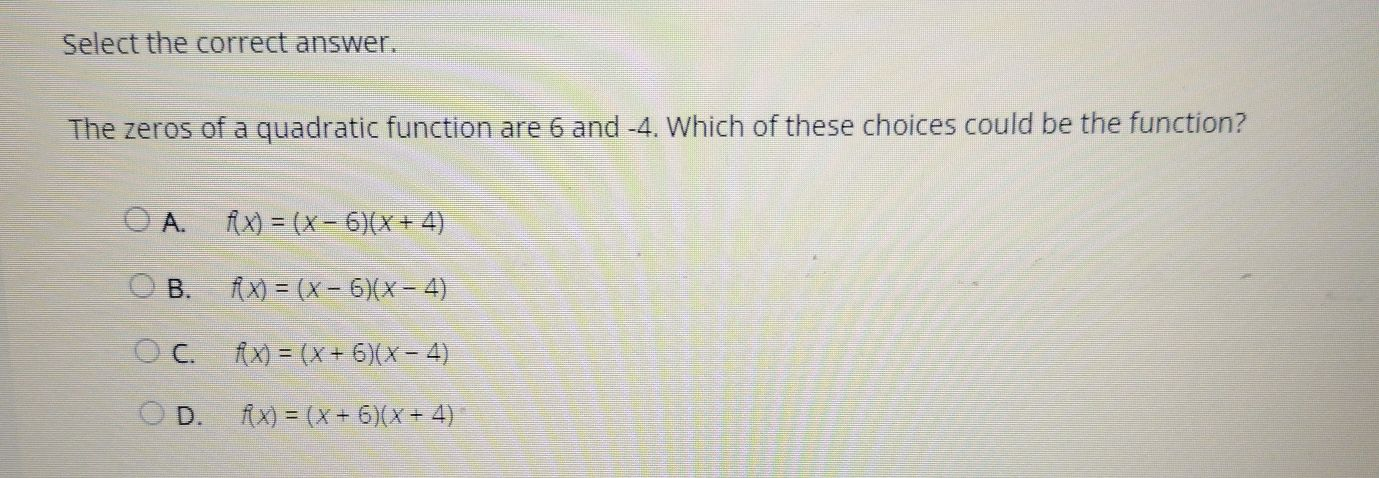### Still have math questions?

Algebra
QuestionSelect the correct answer. The zeros of a quadratic function are $$6$$ and $$- 4$$ . Which of these choices could be the function? A. $$f ( x ) = ( x - 6 ) ( x + 4 )$$

B. $$f ( x ) = ( x - 6 ) ( x - 4 )$$

C. $$f ( x ) = ( x + 6 ) ( x - 4 )$$

D. $$f ( x ) = ( x + 6 ) ( x + 4 )$$